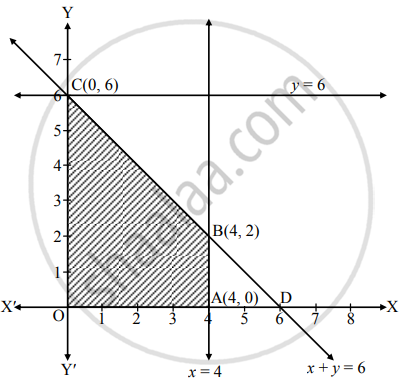Advertisement Remove all ads

# Solve the following L.P.P. by graphical method : Maximize : Z = 11x + 8y subject to x ≤ 4, y ≤ 6, x + y ≤ 6, x ≥ 0, y ≥ 0. - Mathematics and Statistics

Graph

Solve the following L.P.P. by graphical method :

Maximize : Z = 11x + 8y subject to x ≤ 4, y ≤ 6, x + y ≤ 6, x ≥ 0, y ≥ 0.

Advertisement Remove all ads

#### Solution

To draw the feasible region, construct table as follows:

 Inequality x ≤ 4 y ≤ 6 x + y ≤ 6 Corresponding equation (of line) x = 4 y = 6 x + y = 6 Intersection of line with X-axis (4, 0) – (6, 0) Intersection of line with Y-axis – (0, 6) (0, 6) Region Origin side Origin side Origin side

Shaded portion OABC is the feasible region,
whose vertices are O(0, 0), A(4, 0), B and C(0, 6).
B is the point of intersection of the lines x = 4 and x + y = 6Substituting x = 4 in x + y = 6, we get
y = 2
∴ B ≡ (4, 2)
Here, the objective function is Z = 11x + 8y
∴ Z at O(0, 0) = 11(0) + 8(0) = 0
Z at A(4, 0) = 11(4) + 8(0) = 44
Z at B(4, 2) = 11(4) + 8(2) = 44 + 16 = 60
Z at C(0, 6) = 11(0) + 8(6) = 48
∴ Z has maximum value 60 at B(4, 2)
∴ Z is maximum, when x = 4 and y = 2.

Is there an error in this question or solution?
Advertisement Remove all ads

#### APPEARS IN

Advertisement Remove all ads
Advertisement Remove all ads
Share
Notifications

View all notifications

Forgot password?